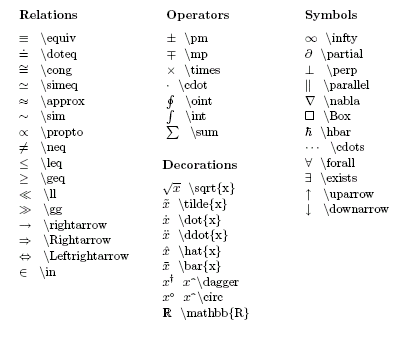## LaTeX for Physicists

To get LaTex running on a windows system,

4. Copy the header to the folder where you will save your document

The LaTeX for Physicists Header has the following features:

• Sets font size to 11pt
• Includes commonly needed packages
• Reduces page margins to 0.75in for more space
• Redefines \maketitle to save space
• Removes page numbers
• Changes enumeration to letters as in physics exercises
• \v{ } makes bold vectors (\v is redefined to \vaccent)
• \uv{ } makes bold unit vectors with hats
• \gv{ } makes bold vectors of greek letters
• \abs{ } makes the absolute value symbol
• \avg{ } makes the angled average symbol
• \d{ }{ } makes derivatives (\d is redefined to \underdot)
• \dd{ }{ } makes double derivatives
• \pd{ }{ } makes partial derivatives
• \pdd{ }{ } makes double partial derivatives
• \pdc{ }{ }{ } makes thermodynamics partial derivatives
• \ket{ } makes Dirac kets
• \bra{ } makes Dirac bras
• \braket{ }{ } makes Dirac brackets
• \matrixel{ }{ }{ } makes Dirac matrix elements
• \div{ } makes a divergence operator (\div is redefined to \divsymb)
• \curl{ } makes a curl operator
• \={ } makes numbers appear over equal signs (\= is redefined to \baraccent)

General LaTeX tips:

• Use "$...$" for inline equations
• Use "$...$" for equations on their own line
• Use "\begin{center} ... \end{center}" to center something
• Use "\includegraphics[width=?cm]{filename.eps}" for images - must compile to dvi then use dvipdfm from a batch file
• Use "\begin{multicols}{2} ... \end{multicols}" for two columns
• Use "\begin{enumerate} \item ... \end{enumerate}" for parts of physics exercises
• Use "\section*{ }" for sections without numbering
• Use "\begin{cases} ... \end{cases}" for piecewise functions
• Use "\mathcal{ }" for a caligraphic font
• Use "\mathbb{ }" for a blackboard bold font

Below is a list of commonly used builtin symbolsLaTeX Symbol Search - search for LaTeX code by its mathematical name
Comprehensive List of Symbols